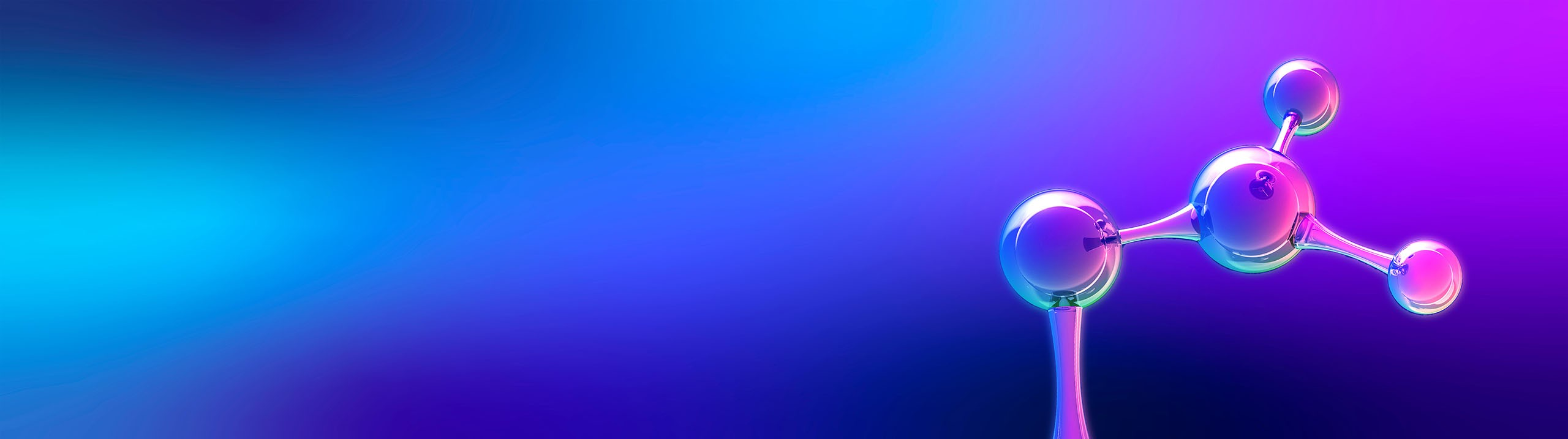Start typing, then use the up and down arrows to select an option from the list.# Organic Chemistry

Learn the toughest concepts covered in Organic Chemistry with step-by-step video tutorials and practice problems by world-class tutors.

17. Aromaticity
1

#### concept

Counting pi electrons1m
Play a video:
in this video, we're gonna become experts on counting pie electrons. So, technically, a pie electron is any electron found within and unhygienic ized P orbital. Okay, so that's the technical definition. However, for the purposes of this video, I think it's just easier if we memorize a few different types of electrons that air pie electrons. And that's going to kind of cover our bases so we can learn that double bonds, radicals and cat ions all contribute different amounts of electrons to pie. Electrons, double bonds and an ions contribute to pie electrons each radicals contribute one pie electron and cat ions because of the fact that they're empty Orbital's you got it. They contribute zero electrons. So simply the job of counting up I electrons is as easy as counting with your fingers. How many electrons you see by counting up with this method? So I'm gonna have you guys do six compounds and figure out the pie electrons for those six. Go ahead and start off with the first one here and use the rule that I gave you to count up all the pie electrons you see. And then we'll go ahead and do the answer
2

#### example

Count the pi electrons present19s
Play a video:
The answer for the first molecule is six. Because I've got to from this double bond I've got to from this told wind, I've also got another two from this old bond. And I've got zero from this cat Ion. You add it all together and you get six pie electrons. Easy move onto the next one.
3

#### example

Count the pi electrons present13s
Play a video:
The answer for this next one was 10 pie electrons because I've got to to to to to that equals 10 pie electrons. Too easy. Let's move on to the next one.
4

#### example

Count the pi electrons present1m
Play a video:
The answer for this next compound was four pi electrons because I had two electrons coming from my double bond. And then I had another two coming from my and I on which he said contributed to pie electrons as well. That would give us four pi electrons total. All right, now, in orderto answer the next three questions, we're gonna have to get a handle on this tricky notation. So if you see a hydrocarbon ring with just a charge in the middle, that means you've got a lazy, organic chemists on your hands. But, hey, this is an acceptable notation, and you do lead to learn what it means. Basically, if you just see a charge in middle, that means that we're gonna assume so I'm just gonna put here. By definition, this means that we're gonna assume alternating double bonds and then the space left over or the carbon left over the Adam left over would possess that charge. So that means that this molecule that five in the ring is actually a shortcut or a shorthand for a five carbon dying with a positive charge on one carbon. So that being said, go ahead and try to figure out how many pilots runs that has, and then use that information to then draw the correct structures of the following two so that you can get the right answers for those as well. You got this.
5

#### example

Count the pi electrons present12s
Play a video:
All right. So the correct answer for this one was for now that we know how to draw it. Because you had 22 and zero giving us a total of four pi electrons. Go ahead and try the next one.
6

#### example

Count the pi electrons present22s
Play a video:
so the correct structure of this molecule was a triangle with simply one double bond and a negative charge in the space afterwards. Because there was Onley enough space for one told one total. This means that this molecule would have a total of four pi electrons. Let's move on to the last question.
7

#### example

Count the pi electrons present42s
Play a video: